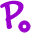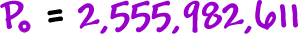Now for some population stuff:

The world's population in 1950 was 2,555,982,611.  In 1970, it was 3,706,601,448.   Find the rate of growth.

* Remember our population equation:Set it up:Can you predict what the world's population will be in 2050?

 Let's use the 1950 figure for our:And we can use the growth rate we just found:1950 to 2050 gives us t = 100...Grab a calculator!So, we predict that there will be 16,392,546,229 people on earth in 2050.